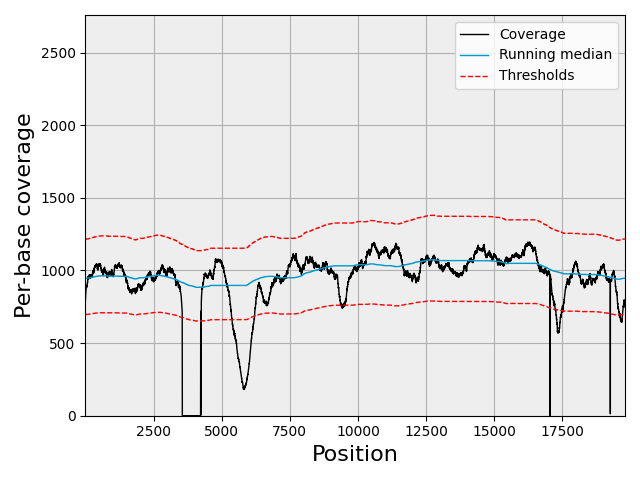# 5.4. Coverage module example

```from sequana import SequanaCoverage, sequana_data

bedfile = sequana_data("JB409847.bed")
```

```gc = SequanaCoverage(bedfile)
```
```0it [00:00, ?it/s]
1it [00:00, 122.55it/s]
```

Select a chromosome (first and only one in that example)

```chrom = gc
print(chrom)
```
```Chromosome length:      19795
Sequencing depth (DOC):                    931.31
Sequencing depth (median):                 988.00
Breadth of coverage (BOC) (percent):        96.60
Coverage standard deviation:        237.15
Coverage coefficient variation:       0.25
```

Compute running median and zscore telling the algorithm that the chromosome is circular.

```chrom.running_median(n=5001, circular=True)
chrom.compute_zscore()
print(chrom.get_centralness())
```
```0.8684516291992928
```

Plotting

```chrom.plot_coverage()
```Total running time of the script: (0 minutes 0.747 seconds)

Gallery generated by Sphinx-Gallery